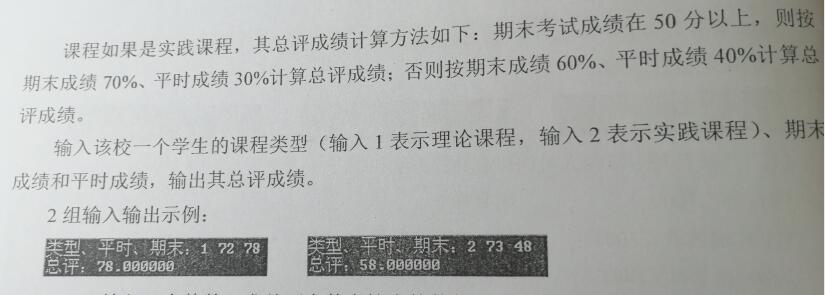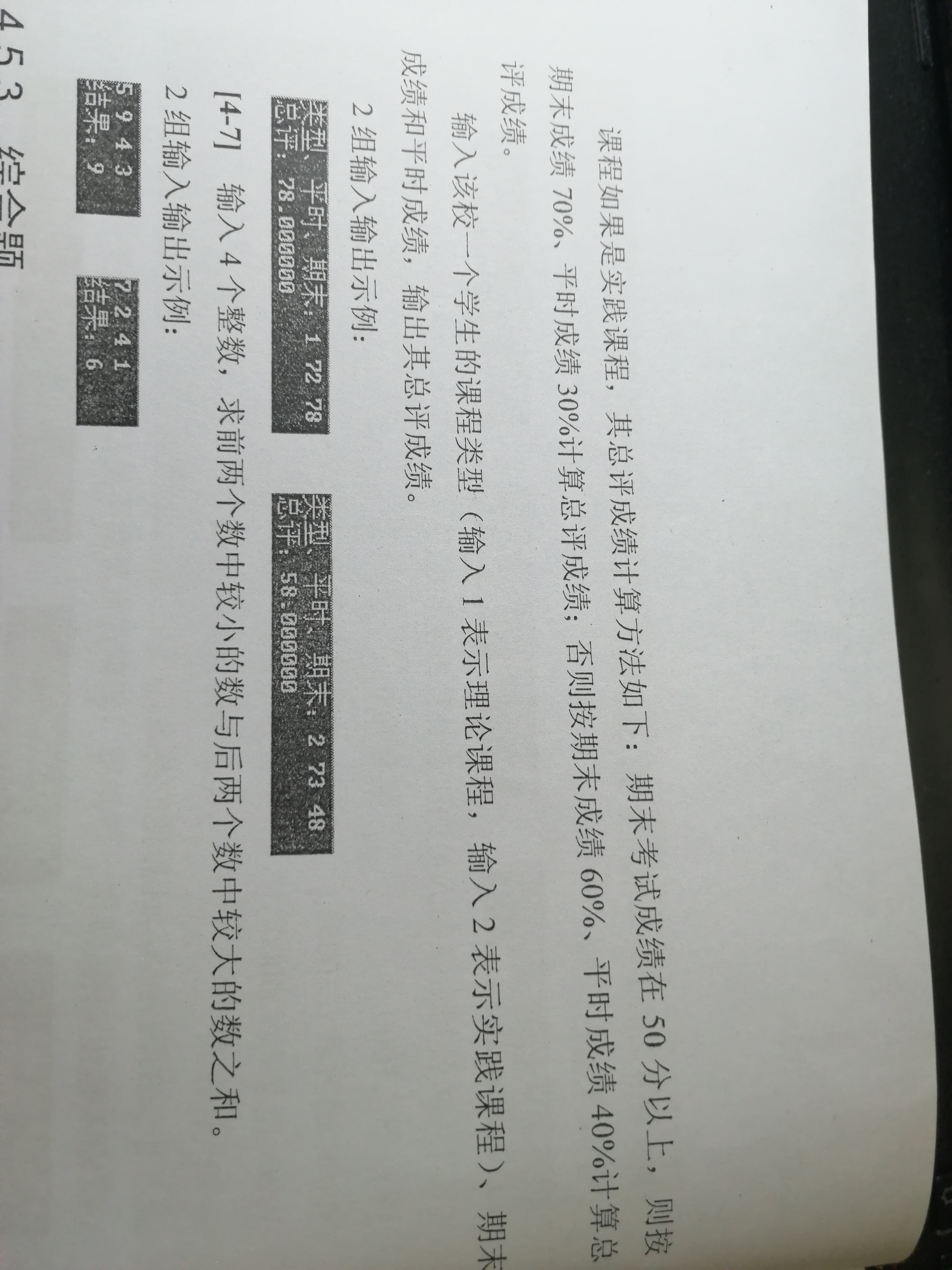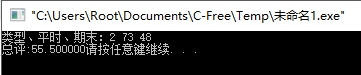2019-09-30 20:42

c语言 请问我的代码为什么测试出来的结果是错的？#include
main()
{
int x,y,z;
float a;

printf("类型、平时、期末：");
scanf("%d%d%d",&x,&y,&z);
if(x=1)
{
if(z>=60)

{

a=z;

}
else

{
a=y*0.3+z*0.7;
}
}
else if(x=2)
{
if(z>=50)
{
a=0.7*z+0.3*y;
}
else
{
a=0.6*z+0.4*y;
}
}

printf("总评:%f",a);
}• 写回答
• 好问题 提建议
• 追加酬金
• 关注问题
• 收藏
• 邀请回答